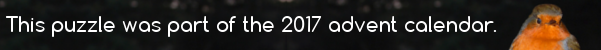mscroggs.co.uk
mscroggs.co.uksubscribe

# Advent calendar 2017

## 19 DecemberPut the digits 1 to 9 (using each digit exactly once) in the boxes so that the products are correct. Today's number is the smallest number that can be made using the digits in the red boxes.
 × × = 90 × × × × × = 84 × × × × × = 48 =64 =90 =63
Tags: numbers, grids

## Archive

Show me a random puzzle
▼ show ▼
© Matthew Scroggs 2019